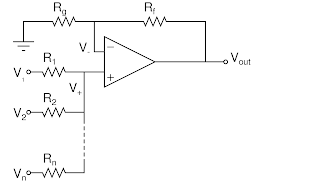## Friday, December 27, 2013

### Non Inverting Summing Amplifier

Here's the circuit:Non Inverting Summing Amplifier, general case

We start by finding the equations for $V_{+}$ and $V_{-}$:
$V_{-} = V_{out} \frac{R_g}{R_g +R_f}$
The currents to the $V_{+}$ node have the same subscripts as the voltages and resistances. We now have a bunch of equations:
$V_{1} - V_{+} = I_{1}R_{1}\\ V_{2} - V_{+} = I_{2}R_{2}$
and so on to:
$V_{n} - V_{+} = I_{n}R_{n}$
We also have
$I_{1} + I_{2} +\cdots + I_{n} = 0$
So by arranging the equations in this form:
$\frac{V_{n} - V_{+}}{R_{n}} = I_{n}$
we get:
$\frac{V_{1} - V_{+}}{R_{1}}+\frac{V_{2} - V_{+}}{R_{2}}+\cdots +\frac{V_{n} - V_{+}}{R_{n}} = 0$
which can be simplified to:
$V_{+}=\frac{V_{1}R_{1}R_{2}\cdots R_{n}/R_1+V_{2}R_{1}R_{2}\cdots R_{n}/R_2+\cdots +V_{n}R_{1}R_{2}\cdots R_{n}/R_n }{R_{1}R_{2}\cdots R_{n}/R_1+R_{1}R_{2}\cdots R_{n}/R_2+R_{1}R_{2}\cdots R_{n}/R_n}$
(Hope I got that notation right...)

Using the golden op amp rules we now set $V_{+}= V_{-}$ and get:
$V_{out} \frac{R_g}{R_g +R_f}=\frac{V_{1}R_{1}R_{2}\cdots R_{n}/R_1+V_{2}R_{1}R_{2}\cdots R_{n}/R_2+\cdots +V_{n}R_{1}R_{2}\cdots R_{n}/R_n }{R_{1}R_{2}\cdots R_{n}/R_1+R_{1}R_{2}\cdots R_{n}/R_2+R_{1}R_{2}\cdots R_{n}/R_n} \Rightarrow \\ V_{out} =\frac{R_g+R_f}{R_g } \frac{V_{1}R_{1}R_{2}\cdots R_{n}/R_1+V_{2}R_{1}R_{2}\cdots R_{n}/R_2+\cdots +V_{n}R_{1}R_{2}\cdots R_{n}/R_n }{R_{1}R_{2}\cdots R_{n}/R_1+R_{1}R_{2}\cdots R_{n}/R_2+R_{1}R_{2}\cdots R_{n}/R_n}$
If we want to, we can simplify this drastically by setting one or both of these equations:
$R_{1} = R_{2} = \ \cdots = R_{n} = R\\ R_{g} = R_{f}$
Will give us:
$V_{out} = 2 \frac{V_{1}+V_{2}+\cdots +V_{n} }{n}$
To get a unity gain summer we can set:
$\frac{R_g+R_f}{nR_g } = 1 \Rightarrow \\ R_f = Rg(n-1)$

That's it. By the way, the schematics I made using xcircuit. It was my first circuit using this tool, but it seems nice enough to try it some more...